Improve math skills of your kids - Learn step-by-step arithmetic from Math games

Math: Unknown - Step-by-step math calculation game for iOS.

Math: Unknown is much more than a math game. It is a step-by-step math calculation game which will teach users how to calculate in the correct order rather than just asking only the final calculated results.

The app consists of four basic arithmetic operations which are addition, subtraction, multiplication and division. In order to get started, users who are new to arithmetic can learn from animated calculation guides showing step-by-step procedures of solving each type of operation. It is also helpful for experienced users as a quick reference.

Generally, addition and subtraction may be difficult for users who just start learning math especially when questions require carrying or borrowing (also called regrouping). The app helps users to visualize the process of carrying and borrowing in the way it will be done on paper. Once users understand how these operations work, they are ready to learn multiplication and division.

For most students, division is considered as the most difficult arithmetic operation to solve. It is a common area of struggle since it requires prior knowledge of both multiplication and subtraction. To help users understand division, the app uses long division to teach all calculation procedures. Relevant multiplication table will be shown beside the question. Users will have to pick a number from the table which go into the dividend. Multiplication of selected number and divisor is automatically calculated, but the users have to do subtraction and drop down the next digit themselves. Learning whole calculation processes will make them master it in no time.

Math: Unknown is a helpful app for students who seriously want to improve arithmetic calculation skills.

Column design and analysis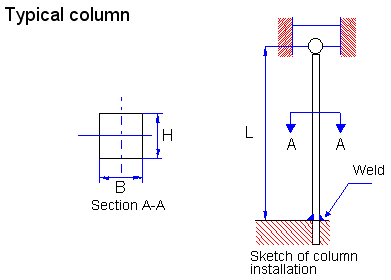In a design situation, the expected load on a column would be known, along with the length required by the application. The designer would then specify the following:
1. The manner of attaching the ends to the structure that affects the end fixity.
2. The general shape of the column cross section (for example, round, square, rectangular, and hollow tube).
3. The material for the column.
4. The design factor, considering the application.
5. The final dimensions for the column.
It may be desirable to propose and analyze several different designs to approach an optimum for the application, so software such as this facilitates the process.
It is assumed that the designer for any given trial specifies items 1 through 4. For some simple shapes, such as the solid round or square section, the final dimensions are computed from the appropriate formula: the Euler formula, or the J. B. Johnson formula. If an algebraic solution is not possible, iteration can be done.
In a design situation, the unknown cross-sectional dimensions make computing the radius of gyration and therefore the slenderness ratio, KL/r, impossible. Without the slenderness ratio, we cannot determine whether the column is long (Euler) or short (Johnson). Thus, the proper formula to use is not known.

We overcome this difficulty by making an assumption that the column is either long or short and proceeding with the corresponding formula. Then, after the dimensions are determined for the cross section, the actual value of KL/r will be computed and compared with Cc. This will show whether or not the correct formula has been used. If so, the computed answer is correct. If not, the alternate formula must be used and the computation repeated to determine new dimensions.
Design factor
Under typical industrial conditions, the design factor of N = 3 is recommended. If the application is very smooth, a value as low as N = 2 may be justified. Under conditions of shock or impact, N = 4 or higher should be used, and careful testing is advised.
Column analysis

The procedure for analyzing straight, centrally loaded columns:
1. For the given column, compute its actual slenderness ratio.
2. Compute the value of Cc.
3. Compare Cc with KL/r. Because Cc represents the value of the slenderness ratio that separates a long column from a short one, the result of the comparison indicates which type of analysis should be used.
4. If the actual KL/r is greater than Cc the column is long. Use Euler's equation:
The equation gives the critical load, Pcr, at which the column would begin to buckle.
An alternative form of the Euler formula is often desirable. Note that:But, from the definition of the radius of gyration, r,
Then
This form of the Euler equation aids in a design problem in which the objective is to specify a size and a shape of a column cross section to carry a certain load.

Notice that the buckling load is dependent only on the geometry (length and cross section) of the column and the stiffness of the material represented by the modulus of elasticity. The strength of the material is not involved at all. For these reasons, it is often of no benefit to specify a high-strength material in a long column application. A lower-strength material having the same stiffness, E, would perform as well.

5. If KL/r is less than Cc, the column is short. Use the J. B. Johnson formula:

Use of the Euler formula in this range would predict a critical load greater than it really is. The J. B. Johnson formula is written as follows: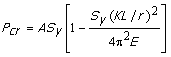The critical load for a short column is affected by the strength of the material in addition to its stiffness, E. As shown in the preceding section, strength is not a factor for a long column when the Euler formula is used.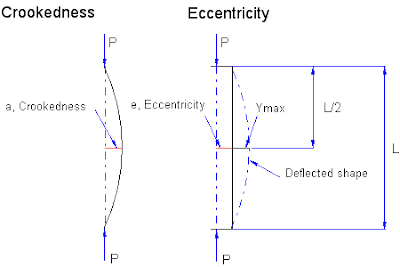An eccentric load is one that is applied away from the centroidal axis of the cross section of the column, as shown in the graphic help entitled “Eccentric column”. Such a load exerts bending in addition to the column action that results in the deflected shape shown in the figure. The maximum stress in the deflected column occurs in the outermost fibers of the cross section at the midlength of the column where the maximum deflection, ymax occurs. Let's denote the stress at this point as sL/2. Then, for any applied load, P,Note that this stress is not directly proportional to the load. When evaluating the secant in this formula, note that its argument in the parentheses is in radians. Also, because most calculators do not have the secant function, recall that the secant is equal to 1/cosine.

For design purposes, we would like to specify a design factor, N, that can be applied to the failure load similar to that defined for straight, centrally loaded columns. However, in this case, failure is predicted when the maximum stress in the column exceeds the yield strength of the material. Let's now define a new term, Py, to be the load applied to the eccentrically loaded column when the maximum stress is equal to the yield strength. The equation then becomesNow, if we define the allowable load to be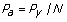or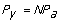this equation becomes

Required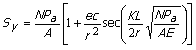This equation cannot be solved for either A or Pa, so an iterative solution is required.
Another critical factor may be the amount of deflection of the axis of the column due to the eccentric load: